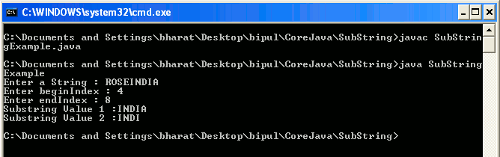Tutorials

# Java Substring

A part of String is called substring. Substrings are also a String. Substring is used to create small strings. Sometimes a programmer needs only a part of String so, how they can find the substring.

A part of String is called substring. Substrings are also a String. Substring is used to create small strings. Sometimes a programmer needs only a part of String so, how they can find the substring.

## Java Substring

A part of String is called substring. Substrings are also a String. Substring is used to create small strings. Sometimes a programmer needs only a part of String so, how they can find the substring. To find the substring in Java there is method named substring(). So, a Java programmer should not to worry about how they can find a part of string from the given String. A programmer can use this method to find out the substring from a given String.

substring() method

This method is overloaded in the String class. Return type of this method is String which is a substring of the current String. This method is written in two forms as :

• substring(int beginIndex) : This method returns a new String called substring of current string. This substring contains the parts of current String started from the beginning index given into the parameter to end of the String. In this substring the value at the beginIndex is always included.
• substring(int beginIndex, int endindex) : This method returns a new String called substring of current string. This substring contains the part of current String started from the beginning index to the specified endIndex-1 i.e. value at the endIndex is always excluded.

Example

Here I am giving a simple example which will demonstrate you about how to find out the substring of current string. In this example we will take input String and the beginIndex and endIndex from user at run time then we will display the substring of current String according to the given beginIndex and endIndex. If a user inputs the string and length of this string is less than the endIndex then the user will be again asked for input the correct length of String and then the required substring will be returned as output.

SubStringExample.java

```import java.util.Scanner;

public class SubStringExample{
public static void main(String args[]){
int beginIndex = 0;
int endIndex = 0;
Scanner scan = new Scanner(System.in);
System.out.print("Enter a String : ");
String str = scan.next();
System.out.print("Enter beginIndex : ");
beginIndex = scan.nextInt();
System.out.print("Enter endIndex : ");
endIndex = scan.nextInt();
if(str.length() < beginIndex || str.length() < endIndex)
{
System.out.println("Enter at least "+endIndex+" characters string :
");
str = scan.next();
System.out.print("Substring Value 1 :" );
System.out.println(str.substring(beginIndex) );
System.out.print("Substring Value 2 :" );
System.out.println(str.substring(beginIndex, endIndex) );
}
else
{
System.out.print("Substring Value 1 :" );
System.out.println(str.substring(beginIndex) );
System.out.print("Substring Value 2 :");
System.out.println(str.substring(beginIndex, endIndex));
}
}
}
```

Output

When you will compile and execute the SubStringExample.java then the output will be as follows :